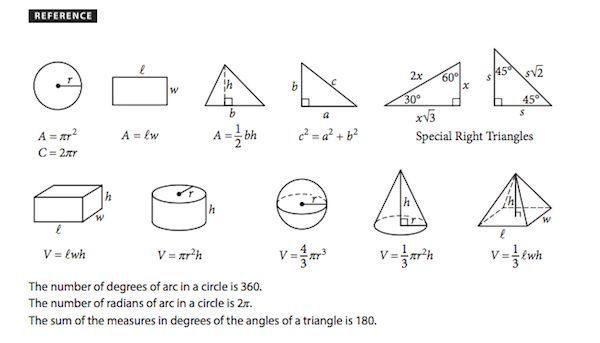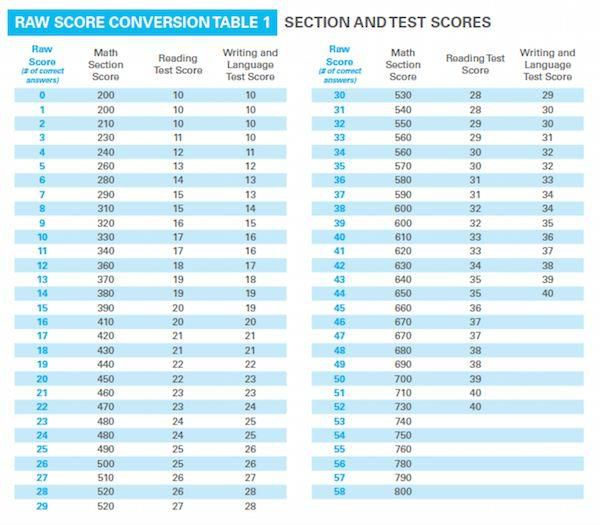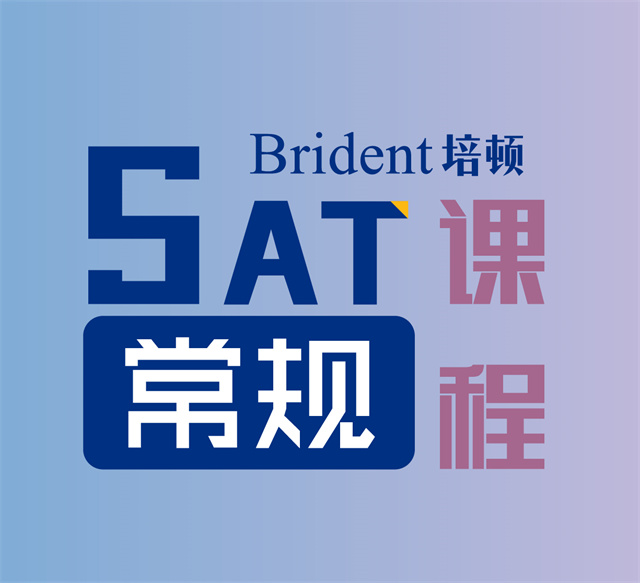## SAT数学基础介绍以及考试内容

1.考试简介

SAT的数学考试部分，总分800分，分成两个部分。

2.syllabus

• Solving linear equations and linear inequalities
• 解一次方程和一次不等式
• Interpreting linear functions
• 解释一次函数的斜率和截距的含义
• Linear inequality and equation word problems
• 关于线性不等式和方程的应用题
• Graphing linear equations
• 一次函数的画图
• Linear function word problems
• 关于一次函数的应用题
• Systems of linear inequalities word problems
• 关于一次不等式组的应用题
• Solving systems of linear equations
• 解二元一次方程组
• 解一元二次方程
• Interpreting nonlinear expressions
• 解释非线性表达式
• Quadratic and exponential word problems
• 关于二次函数和指数函数的应用题
• 有关指数的运算
• Operations with rational expressions and polynomials
• 有理式和多项式的运算
• Polynomial factors and graphs
• 多项式的因式和图像
• Nonlinear equation graphs
• 非线性方程的图像
• 二元二次方程组
• Structure in expressions
• 表达式的构成
• Isolating quantities
• 分离变量（公式变形）
• Functions
• 函数
• Ratios, rates, and proportions
• 比和比例
• Percents
• 百分数
• Units
• 单位换算
• Table data
• 表格类数据分析
• Scatterplots
• 散点图
• Key features of graphs
• 图像的主要特征
• Linear and exponential growth
• 线性增长和指数增长模型
• Data inferences
• 数据分析和推理
• Center, spread, and shape of distributions
• 数据的中心，传播和数据分布的类型
• Data collection and conclusions
• 数据的收集和分析结论
• Volume word problems
• 关于体积的应用题
• Right Triangle word problems
• 关于直角三角形的应用题
• Congruence and similarity
• 全等和相似图形
• Right triangle geometry
• 直角三角形的几何计算
• Angles, arc lengths, and trig functions
• 角，弧长和三角函数
• Circle:Equations and theorems
• 圆的表达式和有关定理
• Complex numbers
• 复数

3.考试公式表4.分数换算表## 猜你喜欢

•很多同学在准备SAT数学的时候，不认识里面的单词导致读不懂题目，刷题效率很慢，也打击了不少考生的信心。所以在备考数学的时候，除了复习之前的知识，还要学习数学专有名词，一切就绪【 查看详情 】
•对于大多数中国考生来说，SAT数学似乎是我们的强项。我们总以为它包含的只是初中阶段的数学知识点。只要我们初中学过数学，再加上适量练习，满分应该不是什么难事。很多同学就是抱着这【 查看详情 】
•SAT的数学考试部分，总分800分，分成两个部分。第一部分，不可以使用计算机，20题（15题选择题，5题填空题），时间25分钟。第二部分，可以使用计算机（包括画图计算机），38题（30题选择【 查看详情 】
•在SAT考试单词准备中，要经历这样一个阶段，也就是针对各种题型来准备单词：有的单词是专门为了做这种题型来准备的，有的单词是专门准备另一种题型的，不同的题型的单词，准备起【 查看详情 】

•SAT一对一高分课程